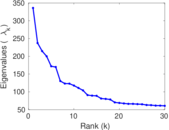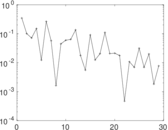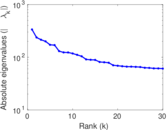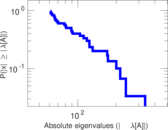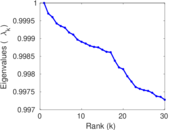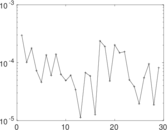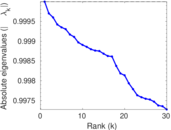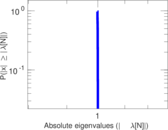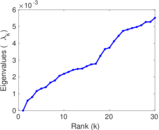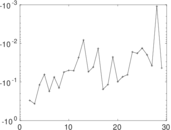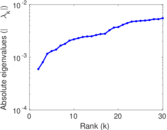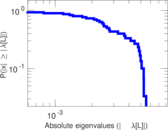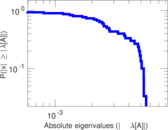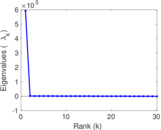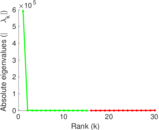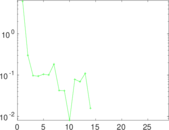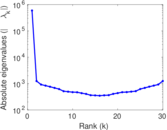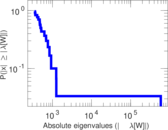# DBpedia countries

This is the bipartite network of entities from DBpedia and their affiliated countries. Each edge in the network connects an entity (such as a person) with a country associated with that person. The edges correspond to the <http://dbpedia.org/ontology/country> relationships in DBpedia. The countries include former countries, empires and kingdoms as well as disputed country-like entities; therefore, the number of country-nodes is much higher than the number of presently existing countries.

 Code `CN` Internal name `dbpedia-country` Name DBpedia countries Data source http://wiki.dbpedia.org/Downloads AvailabilityDataset is available for download Consistency checkDataset passed all tests Category Affiliation network Dataset timestamp 2001 ⋯ 2017 Node meaning Entity, country Edge meaning Affiliation Network formatBipartite, undirected Edge typeUnweighted, multiple edges

## Statistics

 Size n = 592,414 Left size n1 = 590,112 Right size n2 = 2,302 Volume m = 637,134 Unique edge count m̿ = 624,402 Wedge count s = 11,166,368,856 Claw count z = 288,818,329,631,244 Cross count x = 6,941,158,484,979,710,976 Square count q = 66,757,428 4-Tour count T4 = 45,201,226,056 Maximum degree dmax = 111,132 Maximum left degree d1max = 48 Maximum right degree d2max = 111,132 Average degree d = 2.150 98 Average left degree d1 = 1.079 68 Average right degree d2 = 276.774 Fill p = 0.000 459 647 Average edge multiplicity m̃ = 1.020 39 Size of LCC N = 587,255 Diameter δ = 18 50-Percentile effective diameter δ0.5 = 3.685 49 90-Percentile effective diameter δ0.9 = 5.699 25 Median distance δM = 4 Mean distance δm = 4.503 60 Gini coefficient G = 0.535 372 Balanced inequality ratio P = 0.314 907 Left balanced inequality ratio P1 = 0.476 991 Right balanced inequality ratio P2 = 0.052 813 1 Relative edge distribution entropy Her = 0.697 222 Power law exponent γ = 25.392 6 Tail power law exponent γt = 4.511 00 Degree assortativity ρ = −0.088 581 7 Degree assortativity p-value pρ = 0.000 00 Spectral norm α = 335.937 Algebraic connectivity a = 0.000 596 162 Spectral separation |λ1[A] / λ2[A]| = 1.415 23 Controllability C = 588,214 Relative controllability Cr = 0.992 910

## Plots

### Fruchterman–Reingold graph drawing### Degree distribution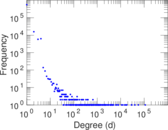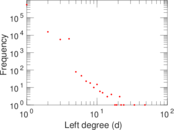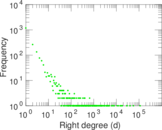### Cumulative degree distribution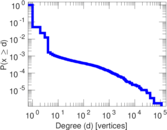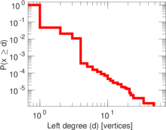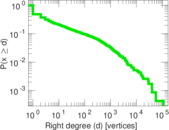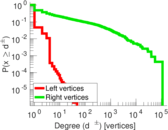### Lorenz curve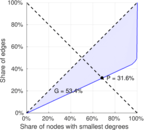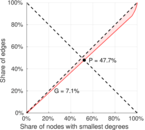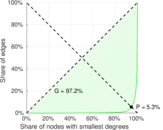### Spectral distribution of the adjacency matrix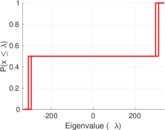### Spectral distribution of the normalized adjacency matrix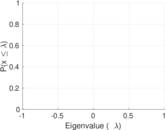### Spectral distribution of the Laplacian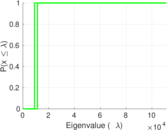### Spectral graph drawing based on the adjacency matrix### Spectral graph drawing based on the Laplacian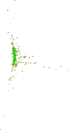### Spectral graph drawing based on the normalized adjacency matrix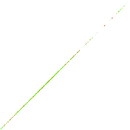### Degree assortativity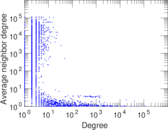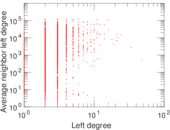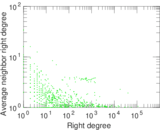### Zipf plot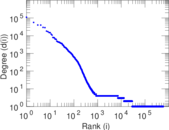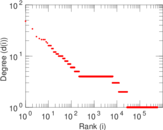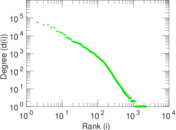### Hop distribution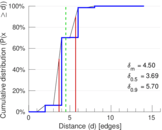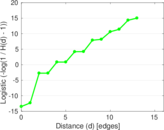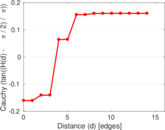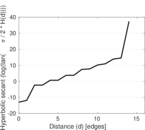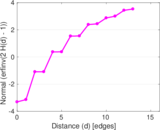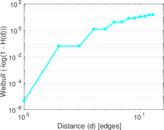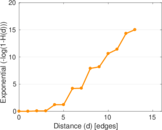### Edge weight/multiplicity distribution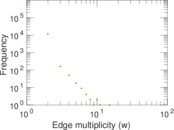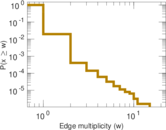### Matrix decompositions plots